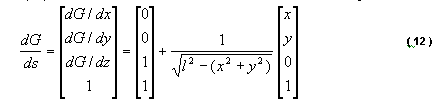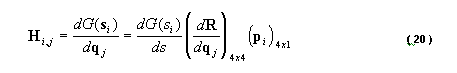Links: [ home | thesis ]

# FAQ: M.S. Thesis: Stewart Platform with Fixed Actuators

## Q1

Excerpt from thesis:From an e-mail:

This is where I have the problem

I would want to know what dg(si)/ds is bcos this is what I have assumed from the equation 20 that dR/dqj is a 4*4 matrix like dR/dx then dR/dy and so on till gama.. given by (numbers) 14,15,16,17,18,19 and.. pi is the position given by equation 5 .. and dg/ds I have taken as given by (number) 12… so according to this then.. we have a 4*1 matrix multiplied by a 4*4 matrix multiplied by a 4*1 matrix which is not possible to get a single value…. So if you culd help me with this it wuld be really of great importance to me……

## A1

```Hij = dG(s[i]) / dq[j]
= {dG(s[i]) / ds}' * { dR / dq[j] } * p[i]
= {    4x1      }  * {    4x4     }  {4x1}
```

{dG/ds} should be transposed{dG/ds} = [1x4], as per eq.(12)
Notice that e.q.(12) bottom-right number should be 0, so that final result in the 4-th row is 1.

My Matlab source code is available at

http://www.cs.columbia.edu/~laza/Stewart/matlab/
look at SPREADME.m, batch02.m, and especially SPForFA.m that calculates forward kinematics for platform with Fixed Actuators.

In SPForFA.m, 3-dimensional matrices were used. For instance, in the code there is R[4x4], q[6x1], then dR/dq=[6x4x4] (equations (14)-(19) each give a 4x4 matrix, which stacked together give 6x4x4) In Matlab code, [6x4x4] is practically arranged as [24x4].

...

You don't have to read the rest of this message: It explains how the Matlab code calcuates forward kinematics.

The variable names are different than in the thesis:

```Thesis -->  Matlab variable
------      -------
b  -->  b
p  -->  p
s  -->  q
l  -->  lengths
R  -->  R
H  -->  G
q  -->  x
dR/dq --> dR
dR/dq*p --> dq
```

Calculating inverse Jacobian G(i,j):

```i :  1..6 = actuator number
j :  1..6 = x,y,z,alpha,beta,gamma = pose coordinate
```
• Calculate dR/dq[j] as given by eq.(14)-(19). You will get 6 matrices, each 4x4. Stack them on top of each other, so you get one large 24x4 matrix.
• You already have p, which is 4x6.
```    x1  x2 ... x6
p = y1  y2     y6
0   0      0
0   0      0
```
• Multiply dR/dq * p = [24x4]*[4x6] = [24x6]. Actually, you have 6 matrices (each per x,y,z,alpha,beta,gamma) stacked on top of each other, each being 4x6, i.e. [xyz0]x[actuators 1..6]
• Calculate dG/ds (eq.(12) derivative of actuator heights wrt. pose). The result is 4x6 (i.e. 6 equations (12) stacked side-by-side).
• Take i-th column from (dR/dq*p). The column has 24 elements. Re-arrange them into a 4x6 matrix, so that the first 4 elements make the first column, the secon 4 elems the second column, etc...
• G(i,:) = i-th row of inverse Jacobian is
```    G(i,:) = transpose(dG/ds[i]) * rearranged(dR/dq*p[i])
```